# Use Custom Constraints in Blending Process

This example shows how to design an MPC controller for a blending process using custom linear input/output constraints.

### Blending Process

A continuous blending process combines three feeds in a well-mixed container to produce a blend having desired concentration. The dimensionless governing equations are: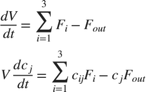where

•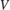is the volume of the mixture inventory in the container.

•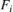is the volume flow rate for feed.

•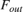is the volume flow rate for the blend exiting the container (the demand from downstream).

•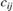is the concentration of constituentin feed.

•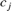is the concentration of constituentin the blend.

•is the time

In this example, there are three feeds,= 1, 2 and 3 and two constituents,= 1 and 2.

The control objectives are to maintain the following values at their setpoints:

• the two constituent concentrations in the blend (quality target)

• the volume of the mixture inventory in the container (safety target)

The challenge is that the downstream demand,, and the concentration of constituents in feeds,, can vary from the nominal operating point.

The mixture inventory, the concentration of constituents in the blendare measured, butare unmeasured.

At the nominal operating condition:

• Feed 1 has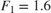,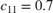and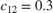• Feed 2 has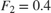,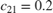and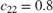• Feed 3 has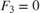,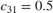and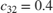In addition, the plant only allows manipulation of the total feed flow rate entering the mixing chamber,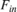, and the individual flow rates of feeds 2,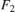, and feed 3,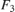. In other words, the flow rate of feed 1,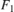, cannot be directly manipulated. However,is indirectly affected by the other three flow rates as follows: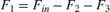Each feed has limited availability imposed by upstream process: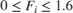### Define Linear Plant Model

The blending process is mildly nonlinear, given how the inventory volume enters the concentration equations, however you can approximate it with a linear model at the nominal steady state. This approach is quite accurate unless the unmeasured feed concentration change. If the change is sufficiently large, the steady-state gains of the nonlinear process change sign, and the closed-loop system can become unstable.

Specify the nominal flow rates for the three input streams and the output stream, or demand. At the nominal operating condition, the output flow rate is equal to the sum of the input flow rates.

Feed = [1.6,0.4,0]; F_out = sum(Feed); 

Define the nominal constituent concentration for the input feeds, where cin(i,j) represents the concentration of constituent j in feed i.

cin = [0.7 0.3;0.2 0.8;0.5 0.4]; 

Define the nominal constituent concentration in the blend.

cout = Feed*cin/F_out; 

The nominal volume of the mixture inventory is 2

V = 2; 

Create a state-space model with feed flows F1, F2, and F3 as inputs

A = [0 0 0; 0 -F_out/V 0; 0 0 -F_out/V]; % 3 states are V, c1 and c2 Bu = [1 1 1; cin(:,1)'/V; cin(:,2)'/V]; % 3 inputs are F1, F2, F3 

Since, as described above, the plant allows manipulation of the total feed rate as well as feeds 2 and 3 (with no direct control of feed 1), change the MV definition from [F1, F2, F3] to [F_in, F2, F3] using equation F1 = F_in - F2 - F3

Bu = [Bu(:,1), Bu(:,2)-Bu(:,1), Bu(:,3)-Bu(:,1)]; % 3 inputs are F_in, F2, F3 

Add the measured disturbance, the blend demand, as the 4th model input.

Bv = [-1; -cout'/V]; B = [Bu Bv]; % 4 inputs are F_in, F2, F3, F_out 

Define all of the states as measurable and no direct feedthrough.

C = eye(3); D = zeros(3,4); 

Construct the linear plant model.

Model = ss(A,B,C,D); Model.InputName = {'F_in','F_2','F_3','F_out'}; Model.InputGroup.MV = 1:3; Model.InputGroup.MD = 4; Model.OutputName = {'V','c_1','c_2'}; 

### Create the MPC Controller

Specify the sample time, prediction horizon, and control horizon for the controller.

Ts = 0.1; p = 10; m = 3; 

Create the controller.

mpcobj = mpc(Model,Ts,p,m); 
-->"Weights.ManipulatedVariables" is empty. Assuming default 0.00000. -->"Weights.ManipulatedVariablesRate" is empty. Assuming default 0.10000. -->"Weights.OutputVariables" is empty. Assuming default 1.00000. 

The outputs are the inventory volume, y(1), and the constituent concentrations, y(2) and y(3). Specify nominal values for all outputs.

mpcobj.Model.Nominal.Y = [V cout(1) cout(2)]; 

Specify the nominal values for the manipulated variables, u(1), u(2) and u(3), and the measured disturbance, u(4).

mpcobj.Model.Nominal.U = [sum(Feed) Feed(2) Feed(3) F_out]; 

Specify output tuning weights. To pay more attention to controlling the concentration of the two constituenta, use larger weights for the second and third outputs.

mpcobj.Weights.OV = [1 5 5]; 

Specify the hard bounds (physical limits) on feed 2 and 3, which are manipulated variables.

mpcobj.MV(2).Min = 0; mpcobj.MV(2).Max = 1.6; mpcobj.MV(3).Min = 0; mpcobj.MV(3).Max = 1.6; 

### Specify Mixed Constraints

We cannot directly specify the lower and upper bounds of feed 1 flow rate like others because it is not a MV. Instead, we have to implement the following inequality constraint as a custom input/output constraint in MPC.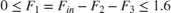Specify this hard constraint in the form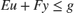.

E = [-1 1 1; 1 -1 -1]; F = [0 0 0; 0 0 0]; g = [0;1.6]; 

Set the custom constraints in the MPC controller.

setconstraint(mpcobj,E,F,g) 

The Simulink model contains a nonlinear model of the blending process and an unmeasured disturbance in the constituent 1 feed concentration.

The Demand,, is modeled as a measured disturbance. The operator can vary the downstream demand value, and the signal goes to both the process and the controller.

The model simulates the following scenario:

• At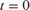, the process is operating at the nominal operating point.

• At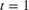, the demand decreases from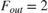to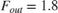.

• At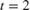, there is a change in the concentration of constituents in feed 1, from [0.7 0.3] to [0.6 0.4].

Open and simulate the Simulink model.

mdl = 'mpc_blendingprocess'; open_system(mdl) sim(mdl) % Open the scope block windows open_system([mdl '/Inputs']) open_system([mdl '/Outputs']) 
-->Converting model to discrete time. -->Assuming output disturbance added to measured output #2 is integrated white noise. -->Assuming output disturbance added to measured output #3 is integrated white noise. Assuming no disturbance added to measured output #1. -->"Model.Noise" is empty. Assuming white noise on each measured output.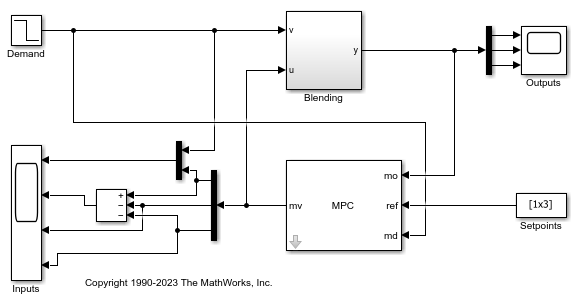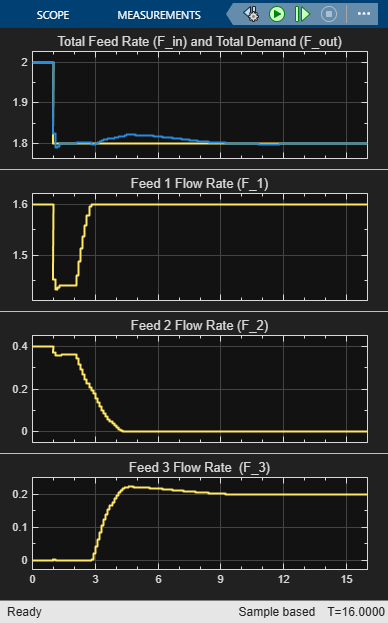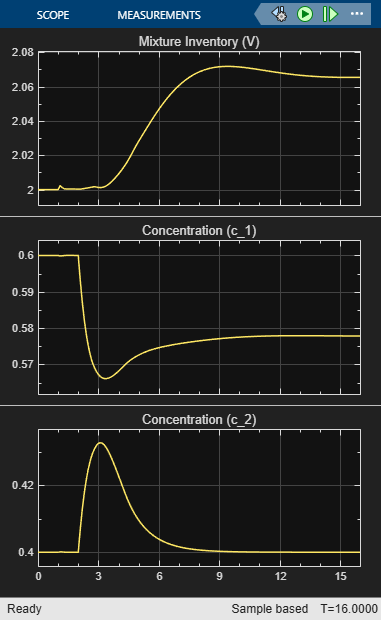In the simulation:

• At time 0, the plant operates steadily at the nominal conditions.

• At time 1, the demand decreases by 10%, and the controller maintains the inventory at its setpoint (V=2).

• At time 2, there is an unmeasured change in the concentration of constituents in feed 1. This disturbance causes a prediction error and a large disturbance in the blend concentration.

The linear MPC controller recovers well and trie to drive the blend concentration back to its setpoint. However, after feed 1 reaches it upper bound (F_1=1.6) enforced by the custom input/output constraint, MPC can no longer maintain zero steady state error due to the feed 1 saturation. With the constraint included, the controller does its best given the physical limits of the system.

bdclose(mdl)